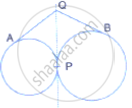Share

# Two Circle Touch Each Other Externally at Point P. Q is a Point on the Common Tangent Through P. Prove that the Tangents Qa and Qb Are Equal. - Mathematics

Course

#### Question

Two circle touch each other externally at point P. Q is a point on the common tangent through P. Prove that the tangents QA and QB are equal.#### Solution

From Q, QA and QP are two tangents to the circle with centre O
Therefore, QA = QP.....(i)
Similarly, from Q, QB and QP are two tangents to the circle with centre O'
Therefore, QB = QP ......(ii)
From (i) and (ii)
QA = QB
Therefore, tangents QA and QB are equal.

Is there an error in this question or solution?